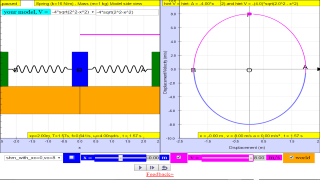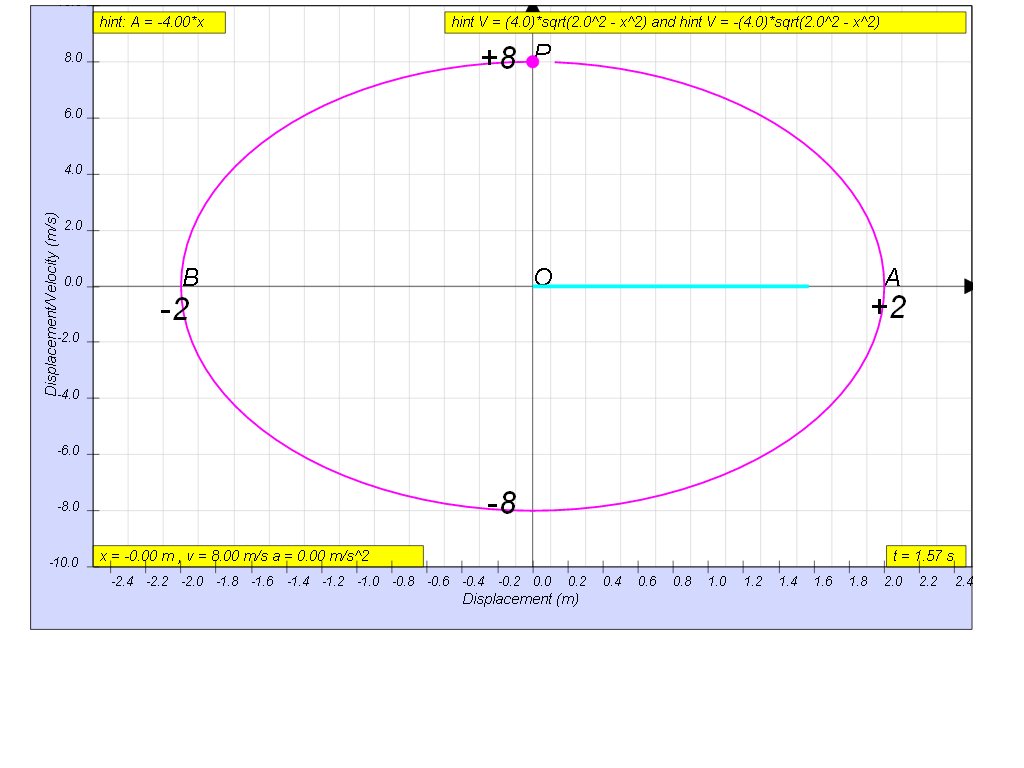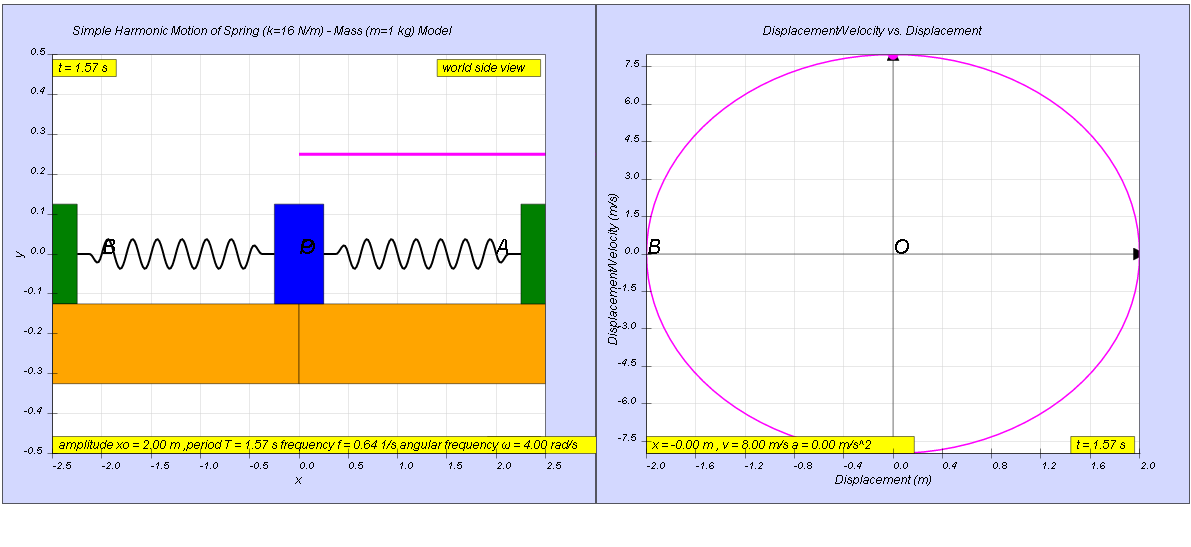## 10.2.10 Example Spring (k=16 N/m) - Mass (m=1 kg) model Velocity versus Position JavaScript HTML5 Applet Simulation ModelSHM16

### 1.2.10 Example

The graph of velocity against displacement of a body is shown below.a)     Explain why there are two values of velocity for zero displacement.
b)    Explain why there are two values of displacement for zero velocity.
c)    Using the maximum value of the velocity, determine the period of oscillation.

[when x =0, v =8(moving right) or -8(moving left),when v =0 , x = -2(at left) or 2(at right),1.6]

##### 1.2.10.1 Suggested Solution:

a)    An oscillating body moves through the equilibrium position (zero displacement) alternately in opposite directions.

b)    An oscillating body has zero velocity when its displacement is maximum which occurs at the  maximum amplitude positions. Hence, there are 2 values of displacement.

c)    vo = xo ω

(8.0) = (2.0) ω

thus

$T=\frac{2\pi }{\omega }$  T  = 1.57 s = 1.6 s   (to 2 s.f.)

### Translations

Code Language Translator Run### Software Requirements

SoftwareRequirements

 Android iOS Windows MacOS with best with Chrome Chrome Chrome Chrome support full-screen? Yes. Chrome/Opera No. Firefox/ Samsung Internet Not yet Yes Yes cannot work on some mobile browser that don't understand JavaScript such as..... cannot work on Internet Explorer 9 and below

### CreditsThis email address is being protected from spambots. You need JavaScript enabled to view it.

### end faq

http://iwant2study.org/lookangejss/02_newtonianmechanics_8oscillations/ejss_model_SHM16/SHM16_Simulation.xhtml